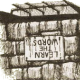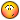# Error: bad argument type - Sum getPoint with getInt variable.

## Recommended PostsHello, I'm building a lisp to add new text based on a standard distance givven by the user. The lisp is:

```(defun c:myFunction()

(setq point (getpoint "Click on start point :"))
(setq qtd   (getint   "\nQuantity of blocks   :"))
(setq dist   (getint  "\nDistance   :"))
(setq x 0  y 0  q 0)

(setq pp point)
(setq x (car pp))

(while (< q qtd)
(setq Text (getstring "Text :"))
(command "text" pp "2" "0" Text )
(setq x (+ pp dist))
(setq q (+ q 1))
)
)```

But when it asks the Text to be entered, I type it but the following error shows up.

(+ PP DIST)

(SETQ X (+ PP DIST))

I think its the type of dist and pp, because one is getint and other is getpoint.

##### Share on other sitesAs a quick fix, maybe change:

```          (setq x (+ pp dist))
```

to:

```          (setq x  (+ x dist))
(setq pp (cons x (cdr pp)))```

##### Share on other sitesThanks so much. It worked!

##### Share on other sitesI have modified your code very slightly to add some error trapping, but not too much so you can understand the changes (the code could still be improved to account for UCS etc).

```(defun c:test ( / pt di qt x str )
(if
(and
(setq pt (getpoint "\nClick on start point: "))
(setq di (getdist  "\nDistance: " pt))
(setq qt (getint   "\nQuantity of blocks: "))
)
(progn
(setq x (car pt))

(repeat qt
(if (not (eq "" (setq str (getstring t "\nText: "))))
(entmake
(list
(cons 0 "TEXT")
(cons 10 pt)
(cons 40 2)
(cons 1 str)
)
)
)
(setq x  (+ x di)
pt (cons x (cdr pt))
)
)
)
)
(princ)
)```

A few notes on the above:

• When prompting for user input, check for null input otherwise other parts of your code expecting this input may fail. All the getXXX (except getstring) will return nil if the user fails to provide an input, so you can use a simple IF statement to check for this input.

• Avoid using the "text" command in LISP, the prompts for this command can vary depending on the textstyle being used, and it is hence unreliable. Instead, consider creating text using entmake as demonstrated by the above code. A reference the DXF codes used can be found here.

• Always localise your variables! To understand why this is important, have a read of this short tutorial.

##### Share on other sites• Avoid using the "text" command in LISP, the prompts for this command can vary depending on the textstyle being used, and it is hence unreliable. Instead, consider creating text using entmake as demonstrated by the above code. A reference the DXF codes used can be found here.

That will always use the STANDARD text style and place on layer 0 - no matter what's current.

I'd also advise using the vla methods for text instead (or modify the height in some cases) ... due to the possibility of text styles being annotative. Or you could omit/calculate the needed text height by multiplying 1/CAnnoScaleValue and the current style's Paper Space Height.

It's a bit of a neither here nor there situation: vla has some hiccups with unicode characters again, so there's no perfect answer to this. So here's a modification which works with the current style / layer / annoscale:

```(defun c:test (/ pt di qt x str [color=red]style lay[/color])
(if
(and
(setq pt (getpoint "\nClick on start point: "))
(setq di (getdist "\nDistance: " pt))
(setq qt (getint "\nQuantity of blocks: "))
)
(progn
(setq x     (car pt)
[color=red]style (entget (tblobjname "STYLE" (getvar "TEXTSTYLE")) '("*"))
lay   (tblsearch "LAYER" (getvar "CLAYER"))[/color]
)

(repeat qt
(if (not (eq "" (setq str (getstring t "\nText: "))))
(entmake
(list
(cons 0 "TEXT")
[color=red](cons 7 (cdr (assoc 2 style)))
(cons 8 (cdr (assoc 2 lay)))[/color]
(cons 10 pt)
[color=red](if (> (cdr (assoc 40 style)) 0)
(if (assoc "AcadAnnotative" (cdr (assoc -3 style)))
(cons 40 (* (cdr (assoc 40 style)) (/ 1.0 (getvar "CAnnoScaleValue"))))
(assoc 40 style)
)
[color=black](cons 40 2)[/color]
)[/color]
(cons 1 str)
)
)
)
(setq x  (+ x di)
pt (cons x (cdr pt))
)
)
)
)
(princ)
)```

##### Share on other sitesIrne, I didn't want to overwhelm the beginner...

##### Share on other sitesIrne, I didn't want to overwhelm the beginner...
Oops! Sorry, I'll do that then## Join the conversation

You can post now and register later. If you have an account, sign in now to post with your account.
Note: Your post will require moderator approval before it will be visible.×   Pasted as rich text.   Paste as plain text instead

Only 75 emoji are allowed.